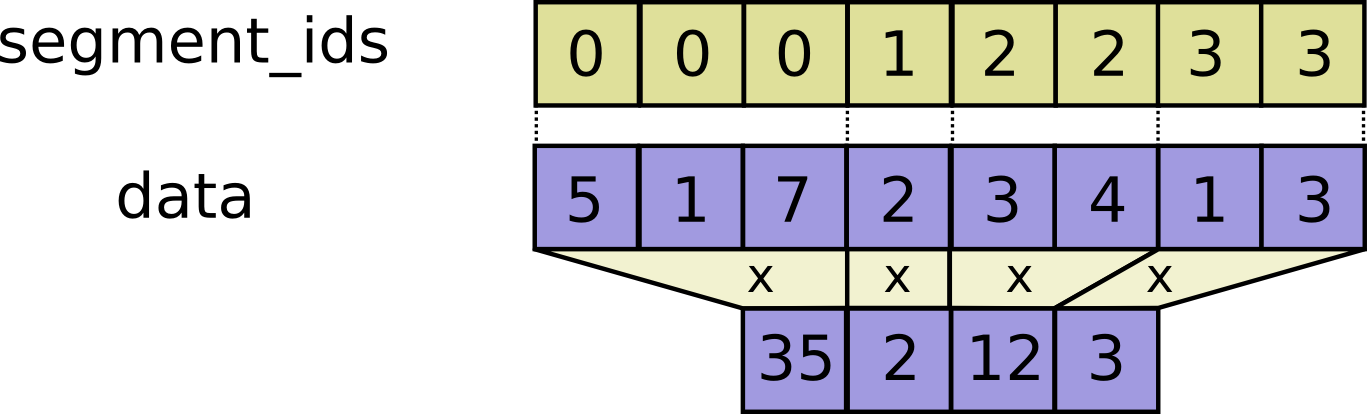# tf.math.segment_prod

Defined in generated file: `python/ops/gen_math_ops.py`

Computes the product along segments of a tensor.

### Aliases:

• `tf.compat.v1.math.segment_prod`
• `tf.compat.v1.segment_prod`
• `tf.compat.v2.math.segment_prod`
``````tf.math.segment_prod(
data,
segment_ids,
name=None
)
``````

Read the section on segmentation for an explanation of segments.

Computes a tensor such that \(output_i = \prod_j data_j\) where the product is over `j` such that `segment_ids[j] == i`.

If the product is empty for a given segment ID `i`, `output[i] = 1`.#### For example:

``````c = tf.constant([[1,2,3,4], [4, 3, 2, 1], [5,6,7,8]])
tf.segment_prod(c, tf.constant([0, 0, 1]))
# ==> [[4, 6, 6, 4],
#      [5, 6, 7, 8]]
``````

#### Args:

• `data`: A `Tensor`. Must be one of the following types: `float32`, `float64`, `int32`, `uint8`, `int16`, `int8`, `complex64`, `int64`, `qint8`, `quint8`, `qint32`, `bfloat16`, `uint16`, `complex128`, `half`, `uint32`, `uint64`.
• `segment_ids`: A `Tensor`. Must be one of the following types: `int32`, `int64`. A 1-D tensor whose size is equal to the size of `data`'s first dimension. Values should be sorted and can be repeated.
• `name`: A name for the operation (optional).

#### Returns:

A `Tensor`. Has the same type as `data`.